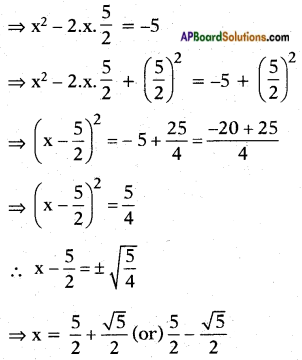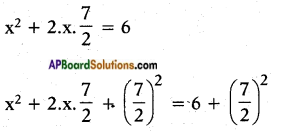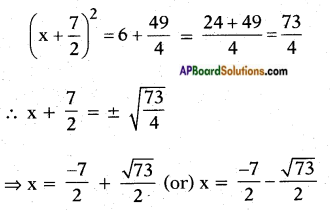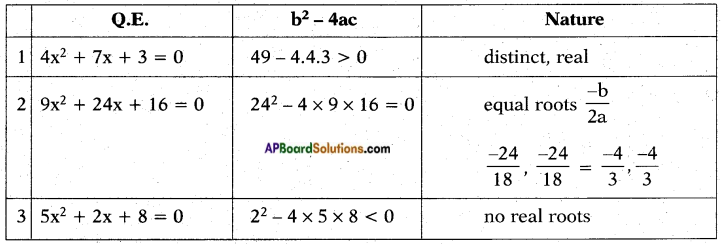# AP SSC 10th Class Maths Solutions Chapter 5 Quadratic Equations InText Questions

## AP State Syllabus SSC 10th Class Maths Solutions 5th Lesson Quadratic Equations InText Questions

AP State Board Syllabus AP SSC 10th Class Maths Textbook Solutions Chapter 5 Quadratic Equations InText Questions and Answers.

### 10th Class Maths 5th Lesson Quadratic Equations InText Questions and Answers

Try this

Question 1.
Check whether the following equations are quadratic or not. (Page No. 102)
i) x2 – 6x – 4 = 0
ii) x3 – 6x2 + 2x – 1 = 0
iii) 7x = 2x2
iv) x2 + $$\frac{1}{\mathbf{x}^{2}}$$ = 2
v) (2x + 1) (3x + 1) = 6(x – 1) (x – 2)
vi) 3y2 = 192
i) x2 – 6x – 4 = 0
ii) x3 – 6x2 + 2x – 1 =0
No. It is not a quadratic equation. [∵ degree is 3]
iii) 7x = 2x2
iv) 2 + $$\frac{1}{\mathbf{x}^{2}}$$ = 2
No. It is not a quadratic equation. [∵ degree is 4]
v) (2x + 1) (3x + 1) = 6(x – 1) (x – 2) No. It is not a quadratic equation, [∵ co-efficient of x2 on both sides is same i.e. 6]
vi) 3y2 = 192

Try this

Question 1.
Verify that 1 and $$\frac{3}{2}$$ are the roots of the equation 2x2 – 5x + 3 = 0. (Page No. 107)
Let the given Q.E. be p(x) = 2x2 – 5x + 3
Now p(1) = 2(1)2 – 5(1) + 3
= 2 – 5 + 3 = 0
∴ 1 is a root of 2x2 – 5x + 3 = 0
also p$$\left(\frac{3}{2}\right)$$ = 2$$\left(\frac{3}{2}\right)^{2}$$ – 5$$\left(\frac{3}{2}\right)$$ + 3
= 2 × $$\frac{9}{4}$$ – $$\frac{15}{2}$$ + 3
= $$\frac{9}{2}$$ + 3 – $$\frac{15}{2}$$
= $$\frac{9+6-15}{2}$$ = 0
∴ $$\frac{3}{2}$$ is also a root of 2x2 – 5x + 3 = 0.

Do this

Question 1.
Solve the equations by completing the square.  (Page No. 113)
i) x2 – 10x + 9 = 0
Given: x2 – 10x + 9 = 0
⇒ x2 – 10x = -9
⇒ x2 – 2.x.5 = -9
⇒ x2 – 2.x.5 + 52 = -9 + 52
⇒ (x – 5)2 = 16
∴ x – 5 = ± 4
∴ x – 5 = 4 (or) x – 5 = -4
⇒ x = 9 (or) x = 1
⇒ x = 9 (or) 1ii) x2 – 5x + 5 = 0
Given: x2 – 5x + 5 = 0
⇒ x2 – 5x = 5iii) x2 + 7x-6 = 0
x2 + 7x – 6 = 0
x2 + 7x = 6Think & Discuss

Question 1.
We have three methods to solve a quadratic equation. Among these three, which method would you like to use 7 Why? (Page No. 115)
If the Q.E. has distinct and real roots, we use factorisation. If Q.E. has no real roots, we use quadratic formula.

Try these

Question 1.
Explain the benefits of evaluating the discriminant of a quadratic equation before attempting to solve it. What does its value signifies?  (Page No. 122)